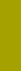﻿ Reynolds' numberSand Texture Sedimentology | Variables

Reynolds' number of sedimenting particle

Variable 5: Logarithmic Reynolds Number, LRE

LRE is a 3-character abbreviation of +log10 Re

In order to quantify hydrodynamic circumstances leading to turbulent or laminar flow, Osborne REYNOLDS (1883) defined a dimensionless ratio between inertia and viscous forces of a flowing fluid as follows:

Re = LV/n,

where L [cm] is a cross sectional diameter of a flow,

V [cm/sec] is flow velocity, and

n [cm2/sec] is kinematic viscosity of the fluid (n is the transcription of the original Greek letter ν).

This ratio became one of the most important numbers in hydrodynamics, used particularly for con­sideration of dynamic similarity of models.

In Reynolds number of sedimenting particle, a relative motion between a solid (particle) and the surrounding fluid assigns the function of the cross sectional flow diameter (L) to the particle size (d). This way, the Reynolds number becomes a significant characteristic of sedimenting particles and of their sedimentation regime. The nature of the Reynolds number as a ratio indicates its suitability for logarithmic scaling.

Because the Reynolds number is a compound (bivariate) variable, it is defined together with a joint variable (size, density or shape). Our SedVar™ program makes it possible to determine an LRE distribution not only for the joint variable PHI (density and SF are taken as constant: RPH distribution), but also for the joint variable density (PHI and SF are taken as constant: RRS distribution), and for the joint variable shape (PHI and density are taken as constant). Therefore, as with the standard PSI (page 5), three LRE versions for three material types can be calculated; the distribution type is identified by File Name Extension according to the joint variable (see also page 21):

 LRE JOINT CONSTANT File Name VARIABLE VARIABLES EXTENSION PHI Rs, SF .RPH Rs PHI, SF .RRS SF PHI, Rs .RSF

The PSI-RPH conversion implies a change of distribution type in order to obtain a compromise between PSI and PHI distribution types (a medium skewing effect between PSI and PHI). The choice of LRE may be justified when the PSI distribution shows the same but opposite skewing as the PHI distribution of the same population.

 RRS distribution has nothing to do with the Rosin-Rammler(-Sperling) frequency distribution. To avoid confusion, for the Rosin-Rammler- (-Sperling) distribution, the more popular shorter abbreviation “RR distribution” only will be used (see also page 3).